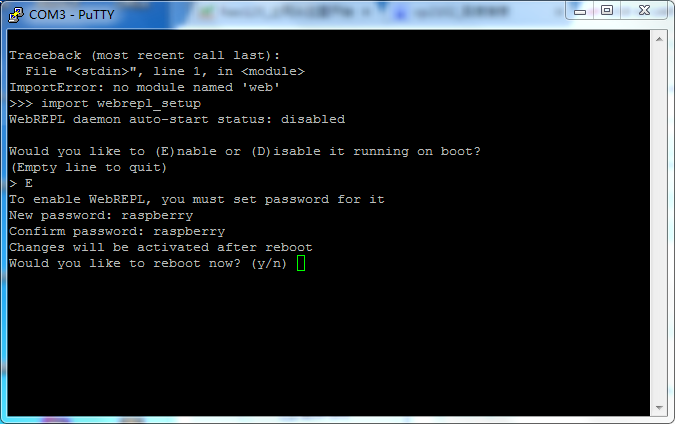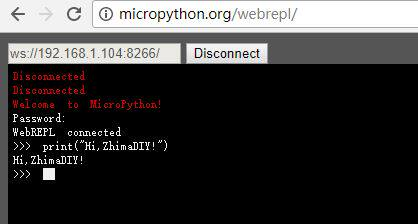# MicroPython:使用WebREPL局域网内远程连接开发板``` def do_connect(): import network sta_if = network.WLAN(network.STA_IF) ap_if = network.WLAN(network.AP_IF) if ap_if.active(): ap_if.active(False) if not sta_if.isconnected(): print('connecting to network...') sta_if.active(True) sta_if.connect('MERCURY_D5C8', 'zhimadiy') #wifi的SSID和密码 while not sta_if.isconnected(): pass print('network config:', sta_if.ifconfig()) do_connect() gc.collect() ``````import webrepl webrepl.start()```